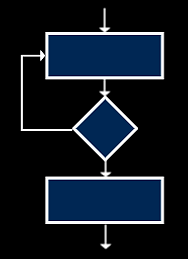top of page

## Programming Constructs

There are three constructs (ideas of programming) that are used to control the flow of a program:

Sequence

Structuring code into a logical, sequential order.Selection

Decision making using if statements.Iteration

Repeating code using for or while loops.## Variables

Variables are used to store data in programs. They can be changed as the program runs.

A variable has two parts - the data value such as "Emily" and an identifier such as First_Name

An efficient program will use variables with sensible identifiers that immediately state their purpose in the program.

Using variable names like 'TotalNum' and 'Profit' rather than 'num1' and 'num2' mean that other programmers will be able to work out the purpose of the code without the need for extensive comments.

## Local & Global Variables

Large programs are often modular - split into subroutines with each subroutine having a dedicated purpose.

Local variables are declared within a specific subroutine and can only be used within that subroutine.

Global variables can be used at any point within the whole program.

• Saves memory - only uses memory when that local variable is needed - global variables use memory whether they are used or not.

• Easier to debug local variables as they can only be changed within one subroutine.

• You can reuse subroutines with local variables in other programs.

• Variables can be used anywhere in the whole program (and in multiple subroutines).

• Makes maintenance easier as they are only declared once.

• Can be used for constants - values that remain the same.

## Constants

π

As specified before, a variable is data that can change in value as a program is being run.

A constant is data that does not change in value as the program is run - it is fixed and remains the same.

An example of a constant in maths programs is pi - it will constantly remain at 3.14159 and never change.

## Comparison Operators

Comparison operators are used to compare two data values. A table of common comparison operators used in programs are below:## Arithmetic Operators

Arithmetic operators are used to mathematically manipulate values.

The most common arithmetic operators are add (+), subtract (-), multiply (*) and divide (/).

Further arithmetic operators are shown below:Modulo division (also known as modulus) reveals the remainder from the last whole number. For example:

9 % 4 = 1 (4 goes into 9 twice (8) with a remainder of 1)Integer division (also known as quotient) reveals the ‘whole number of times’ a number can be divided into another number

9 // 4 = 2 (4 goes into 9 fully, twice)

The symbol ^ represents exponentiation.

For example '2^3 = 8' as it means '2³ = 8'.

## Logical Operators

Logical operators typically use TRUE and FALSE values which is known as Boolean.## Questo's Questions

2.1 - Programming Fundamentals:

Programming Constructs

1. Describe and draw a diagram for the 3 programming constructs. 

Variables

1. What is the difference between local and global variables? 

2. Describe two advantages of using local variables. 

3. Describe two advantages of using global variables. 

4. What is a constant? Give an example. 

bottom of page